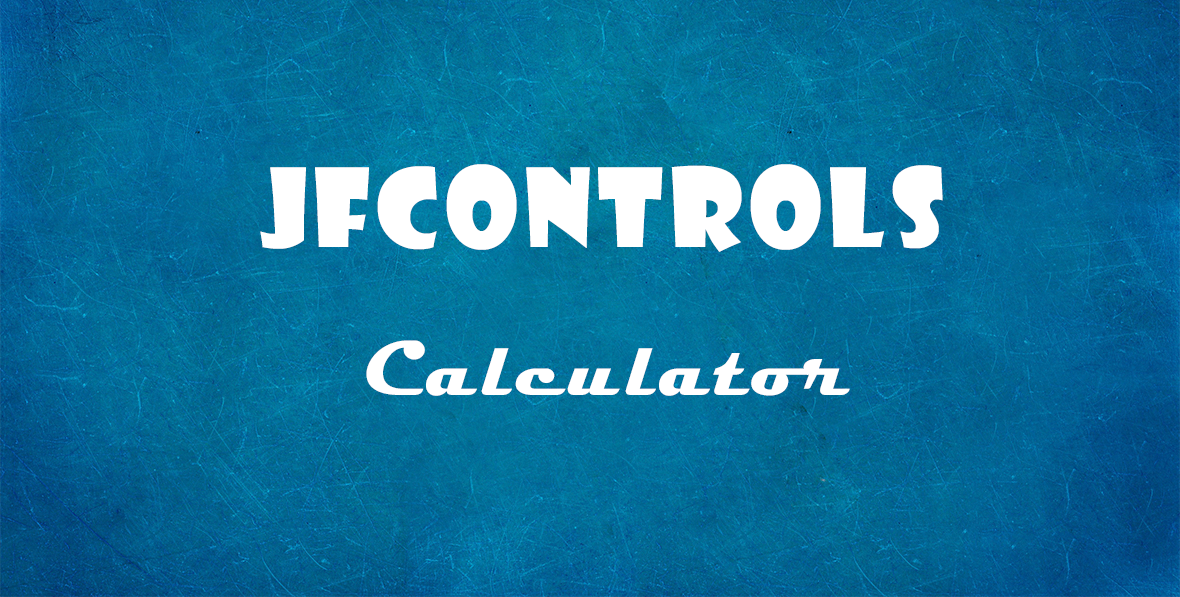Select Page

#### JfControls

How can I execute the calculator with source code?

#### 0 Comment(s)#### Written by Jose AntonioWith the last version of JfControls the calculator should be executed as follows:

``````procedure Calculator;
var
ANumber: Extended;
begin
ANumber := 1000;
JfCApp.Calculator(2,ANumber);
end;``````

With older versions, you will need to do the following:

``````procedure Calculator;
var
ANumber: Double;
begin
ANumber := 1000;
JfCApp.Calculator(2,ANumber);
end;``````

where 2 is the number of decimals.